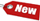Browse Accounting Lessons HereAccounting Terms & DefinitionsAccounting for Merchandising ActivitiesDebits and Credits (Double Entry Accounting)Business Valuation FormulasTime Value of Money & Present/Future ValuesComplex Debt & Equity InstrumentsCommon Stock & Shareholder's EquityAccounting & Finance RatiosValuing Common StockCorporate Income TaxesLower of Cost or Market (LCM) & Inventory ValuationChart of Accounts & BookkeepingBonds Payable & Long Term LiabilitiesCapital AssetsGAAP, Accrual & Cash Accounting, Information Commodity, Internal Controls & MaterialityWhat category of browser are you on this website? Accounting student (homework help) Finance professor (university research) Accounting manager (at work) Other Explore Careers in Accounting and FinanceVisit our section on Careers in Accounting & Finance to explore vast opportunities in this industry.

Chapter 4.9® - Determining the Discount Rate using Basic Present Value equation & Finding the Number of Accounting Periods

We can determine the discount rate in an investment by using the basic present value equation. The equation is;

 PV = FVt / (1 + r)t

There are 4 parts to this equation: the present value (PV), the future value (FVt), the discount rate (r) and life of the investment (t). If we are given 3 of these factors, we can easily find the fourth one.

Let’s consider an example where we have \$1,000 and we are offered to put it in investments that will double this money in 8 years, what is the rate of return we are getting? In other words, we are trying to find the rate of return given the present value, future value and # of periods (years). To calculate the discount rate, let’s plug these numbers in to an equation:

 PV = FVt / (1 + r)t \$1000 = \$2000 / ( 1 + r)8 ( 1 + r)8 = \$2000 / \$1000 ( 1 + r)8 = \$2

To solve this equation, we must take a 1/8th root from each side. To take the 8th root from each side, on your calculate, press 2 yx 0.125 = ?
Note: We come up with 0.125 because 1 / 8 equal 0.125

The 8th root turns out to be about 1.09, which implies that the rate of return is 9%

What if we do this calculation using our financial calculator? Here are the variables

 N = 8 I/Y = ? PV = \$-1,000 PMT = \$0 FV = \$2,000 2ND I/Y P/Y = 1 C/Y = 1 CPT & I/Y = 9.05%

Notice both methods bring us 9% as the rate of return.

Finding the Number of Periods

Suppose we need to buy a BMW 745i for \$60,000 and currently we have \$25,000 on hand. If we can earn 7% rate of return on this principal balance, how much time do we need to wait before we have \$60,000? This answer involves solving for the last variable in the basic present value equation, number of periods. Let’s manipulate the present value equation to derive our answer.

 PV = FVt / (1 + r)t \$25,000 = \$60,000 / (1 + 0.07)t (1 + 0.07)t = \$60,000 / \$25,000 1 .07t = 2.4 t x 1.07 = 2.4 t = 2.4 / 1.07 t = 2.24

Next we look at 2.24 in the Future Value of a Lump Sum table in the 7% column. The answer is 12 years, which is close to what our financial calculator will tell us.

If we do this calculation using our financial calculator, here are the numbers plugged in:

 N = ? I/Y = 7% PV = \$-25,000 PMT = \$0 FV = \$60,000 2ND I/Y P/Y = 1 C/Y = 1 CPT & N = 12.9 years

As you can see from our answer, we would need to wait 12.9 years for our original principal of \$25,000 to grow to \$60,000 at a rate of 7% interest compounded annually.